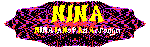#NINA's MiniNina Applet

It is easy to embed Nina figures on your own web page, even if you know nothing about Java. First, download the MiniNina applet archive file, available as a tar or a zip file, and untar/unzip it. It consists of five Java class files. These files should be put in the same directory as the HTML file you want the applet to appear on. Use the APPLET tag to include the MiniNina.class. The following parameters are available:

background
What color to use as the backdrop. Acceptable values are "black" and "white". Default is "black". No complaints -- that is twice as many choices as Henry Ford gave for the Model T!
max_lines
The maximum number of lines that will appear in a figure. Default value is 2000.
max_colors
The maximum number of colors to use per figure. The default value is 4.
show_animation
Should the animated line-drawing be shown? Acceptable values are "true" and "false". Default is "true".
line_delay
If show_animation is true, how many milliseconds to pause between each line drawn. The default value is 5.
auto_draw
Should new figures automatically be drawn, or should Nina only redraw on mouse-click? Acceptable values are "true" and "false", the default is "true".
figure_delay
If auto_draw is true, how many seconds to pause before drawing a new figure. The default is 5.
seed
An integer to be used as a seed to the random number generator. If this is not provided the system clock is used as the seed. But if you have more than one Nina on the same page, they are likely to get the same seed, and hence draw the same sequence of figures. So if you have multiple Ninas on a page, it is best to give them each their own seed (Note that a given seed will not generate the same figures each time the applet is loaded).

To make this a bit more clear, here are a couple examples of use of the APPLET tag, along with the results they produce:

```<APPLET CODE="MiniNina.class"
NAME="Nina"
WIDTH=300
HEIGHT=300>
<PARAM name="max_lines"    value="500">
<PARAM name="line_delay"   value="5">
<PARAM name="figure_delay" value="5">
<PARAM name="seed"         value="1">
<PARAM name="background"   value="white">
</APPLET>
```

```<APPLET CODE="MiniNina.class"
NAME="Nina"
WIDTH=150
HEIGHT=150>
<PARAM name="max_lines"    value="200">
<PARAM name="line_delay"   value="40">
<PARAM name="figure_delay" value="0">
<PARAM name="seed"         value="2">
<PARAM name="background"   value="black">
</APPLET>
```